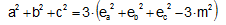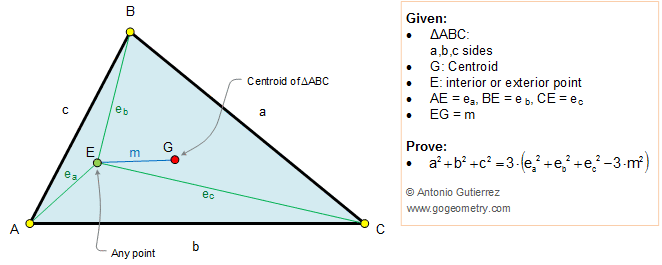Problem 255. Triangle, Centroid, Vertices, Interior/Exterior Point, Distances, Squares The figure shows a triangle ABC. G is the centroid G (point of concurrency of the triangle's medians) and E is any point. If BC =a, AC = b, AB = c, AE = ea, BE = eb, CE = ec, and EG = m, prove that. View or post a solution.Home | Sitemap | Geometry | Problems | 251-260 | View or post a solution